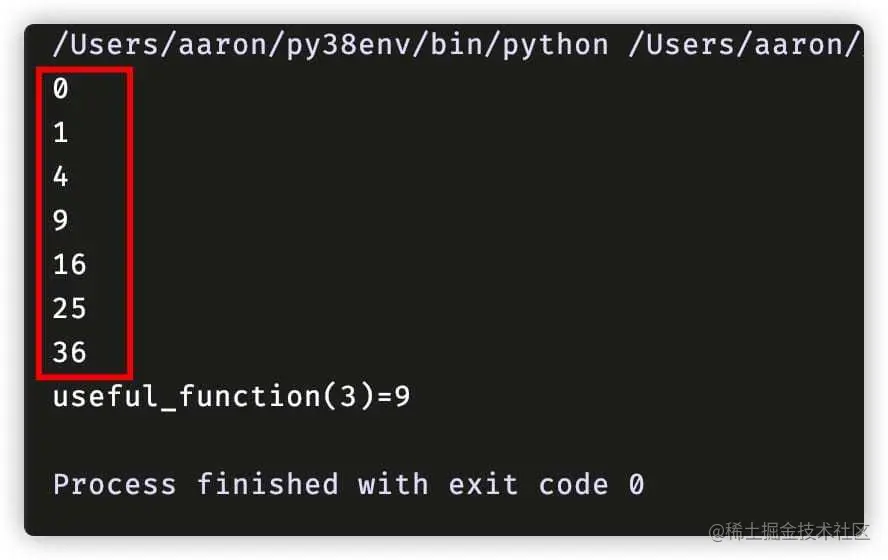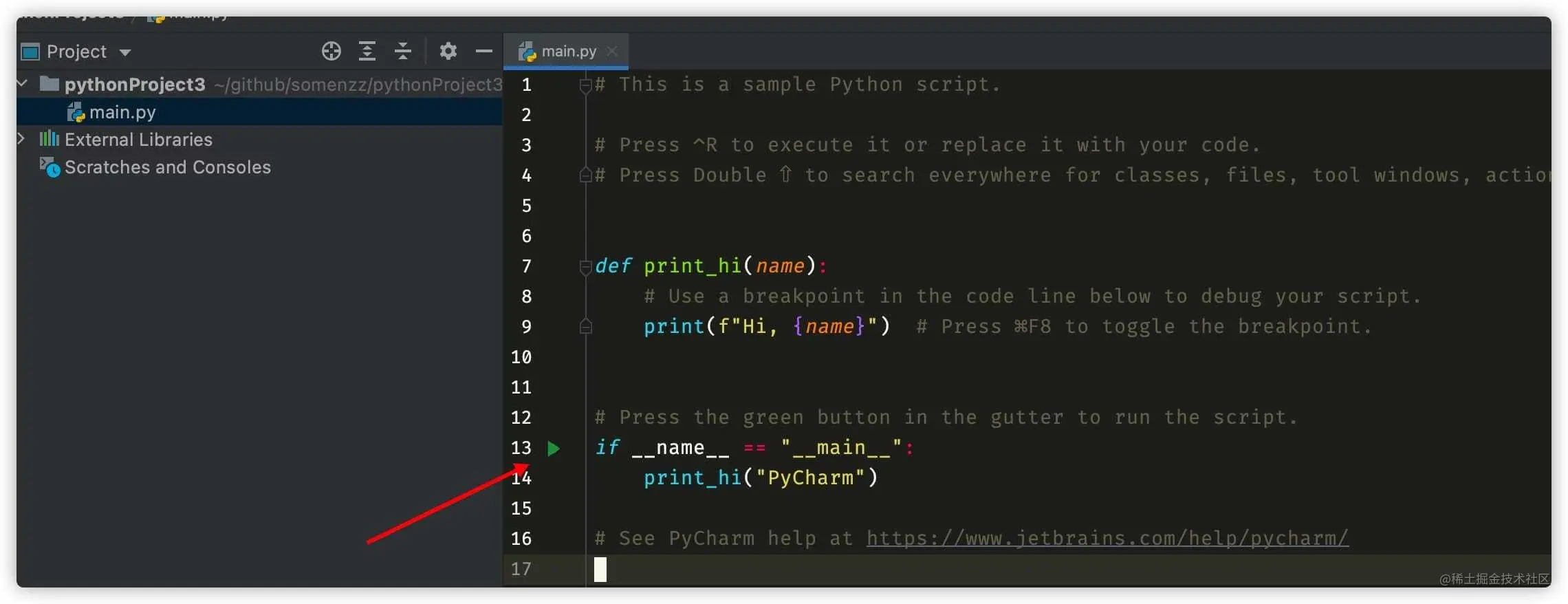# When writing Python scripts, be sure to add this

2022-01-30 18:52:52 somenzz

I found that many friends wrote Python The script is very casual , Or no function , Or functions are defined everywhere , Anyway, I can't see where the first line of code to be executed is at first sight , Such scripts are poorly readable , And easy to hide bug, in addition , This is not Python Community recommended practices , It's easy to solve this problem , When we write Python Script time , Make sure to add this ：

``````def main():
# do something
print("do something.")

if __name__ == "__main__":
main()
Copy code ``````

You may object ： I can write as much as I like , Why listen to you , Write more `if __name__...`?

Don't worry. , Let me say three reasons .

## First of all , It makes Python The role of the document is more clear

First of all, understand `__name__` The role of , When the script is directly Python When the interpreter executes , Its value is "main", When it is used by others Python Program import When , Its value is the corresponding Python Script name , Can be in Python The interpreter verifies , Suppose there is a some_script.py It reads as follows ：

``````print("some_script.py")
print(__name__)
Copy code ``````

stay Python The interpreter imports ：

``````* vim some_script.py
* python
Python 3.8.5 (v3.8.5:580fbb018f, Jul 20 2020, 12:11:27)
[Clang 6.0 (clang-600.0.57)] on darwin
>>> import some_script
some_script.py
some_script
>>>
Copy code ``````

You can see ,`__name__` The value is Python The filename of the script some_script.

in other words `if __name__ == "__main__":` The following code is import It won't run when .

I understand that ,`if __name__ == "__main__":` It can be used as a flag to distinguish between scripts and Libraries , When we see `if __name__ == "__main__":` when , I think this is a script that can be run directly , When you don't see this line of code , I think this is a library , Can be referenced by other programs ,`Explicit is better than implicit.`, isn't it? ？

Another example ：

If you write one without `if __name__ == "__main__":` Script for , It's called bad_script.py, The contents are as follows ：

``````def useful_function(x):
return x * x

class UsefulClass:
def __init__(self, x):
self.x = x

# You tested it yourself , No problem
for i in range(7):
print(useful_function(i))
Copy code ``````

Someone else wrote useful.py, Quoted your useful_function：

``````from bad_script import useful_function

def main():
print(f'{useful_function(3)=}')

if __name__ == '__main__':
main()
Copy code ``````

I. operation , Unexpected content was found printed , See the red part in the figure below ：For a long time , It was found that it was the output of your script , Do you think others will scold you ？

## second , It makes Python Documents are easier to read , Yes IDE friendly

With `if __name__ == "__main__":` amount to Python The program also has an entry function , We can clearly know where the logic of the program begins （ Of course, we also need to consciously put the starting logic of the program here ）

Actually , This is also PyCharm Recommended Practice , When you create a new project , It creates by default main.py It's like this ：stay `if __name__ == "__main__":` There is also a Green Run button on the far left of the line , Click on it. , The program starts from this line .

Why are many good programming languages , such as C、Java、Golang、C++ There is one. main The entry function ？ I think a very important reason is that the program entry is unified , Easy to read .

## Third 、 In the multi process scenario , Must use if main

For example, you do parallel computing with multiple processes , Wrote such code ：

``````import multiprocessing as mp

def useful_function(x):
return x * x

print("processing in parallel")
with mp.Pool() as p:
results = p.map(useful_function, [1, 2, 3, 4])
print(results)

Copy code ``````

When you run , You will find that the program keeps creating processes , At the same time, they are constantly reporting errors RuntimeError, Even if you Ctrl C You can't terminate the program . And added `if __name__ == "__main__":` The program will proceed as expected ：

``````import multiprocessing as mp

def useful_function(x):
return x * x

if __name__ == '__main__':
print("processing in parallel")
with mp.Pool() as p:
results = p.map(useful_function, [1, 2, 3, 4])
print(results)
Copy code ``````

Why is that ？

In fact, I understand Hu ,Python Multiple programs start multiple Python Resolver , Every Python The interpreter will import your script , Copy a copy of global variables and functions to the child process , If you have the `if __name__ == "__main__":`, Then the code behind it will not be import, It will not be repeated . otherwise , The code that creates multiple processes will be import, It will be executed , So as to create sub processes infinitely recursively ,Python3 Will be submitted to the RuntimeError, The order is to create the process first , Then report the wrong , So there will be a constant creation process , Keep reporting mistakes ,Ctrl C Can't stop the phenomenon , Can only kill Drop the whole terminal . Here is a Official explanation

## Last words

`if __name__ == "__main__":` Although not mandatory , But for these three reasons , I strongly recommend that you do this , It is Python Community agreement , Corresponding Python zen ： Clarity is better than obscurity . just as `_` As a variable name, it means to tell the person reading the code ： This variable is not important , I won't use it later . When you see Python The script has `if __name__ == "__main__":` when , You realize that , This is an executable script , When imported by other programs , This part of the code will not be executed , In a multi process program , It's a must .

If there is a harvest , Welcome to thumb up 、 Focus on 、 Looking at , Thank you for reading .